# Model Loss Given Default

This example shows how to fit different types of models to loss given default (LGD) data. This example demonstrates the following approaches:

For all of these approaches, this example shows:

• How to fit a model using training data where the LGD is a function of other variables or predictors.

• How to predict on testing data.

The Model Comparison section contains a detailed comparison that includes visualizations and several prediction error metrics for of all models in this example.

The Regression, Tobit, and Beta models are fitted using the `fitLGDModel` function from Risk Management Toolbox™. For more information, see Overview of Loss Given Default Models.

### Introduction

LGD is one of the main parameters for credit risk analysis. Although there are different approaches to estimate credit loss reserves and credit capital, common methodologies require the estimation of probabilities of default (PD), loss given default (LGD), and exposure at default (EAD). The reserves and capital requirements are computed using formulas or simulations that use these parameters. For example, the loss reserves are usually estimated as the expected loss (EL), given by the following formula:

$\mathrm{EL}=\mathrm{PD}*\mathrm{LGD}*\mathrm{EAD}$.

Practitioners have decades of experience modeling and forecasting PDs. However, the modeling of LGD (and also EAD) started much later. One reason is the relative scarcity of LGD data compared to PD data. Credit default data (for example, missed payments) is easier to collect and more readily available than are the losses ultimately incurred in the event of a default. When an account is moved to the recovery stage, the information can be transferred to a different system, loans can get consolidated, the recovery process may take a long time, and multiple costs are incurred during the process, some which are hard to track in detail. However, banks have stepped up their efforts to collect data that can be used for LGD modeling, in part due to regulations that require the estimation of these risk parameters, and the modeling of LGD (and EAD) is now widespread in industry.

This example uses simulated LGD data, but the workflow has been applied to real data sets to fit LGD models, predict LGD values, and compare models. The focus of this example is not to suggest a particular approach, but to show how these different models can be fit, how the models are used to predict LGD values, and how to compare the models. This example is also a starting point for variations and extensions of these models; for example, you may want to use more advanced classification and regression tools as part of a two-stage model.

The three predictors in this example are loan specific. However, you can use the approaches described in this example with data sets that include multiple predictors and even macroeconomic variables. Also, you can use models that include macroeconomic predictors for stress testing or lifetime LGD modeling to support regulatory requirements such as CCAR, IFRS 9, and CECL. For more information, see Incorporate Macroeconomic Scenario Projections in Loan Portfolio ECL Calculations.

### LGD Data Exploration

The data set in this example is simulated data that captures common features of LGD data. For example, a common feature is the distribution of LGD values, which has high frequencies at `0` (full recovery), and also many observations at `1` (no recovery at all). Another characteristic of LGD data is a significant amount of "noise" or "unexplained" data. You can visualize this "noise" in scatter plots of the response against the predictors, where the dots do not seem to follow a clear trend, and yet some underlying relationships can be detected. Also, it is common to get significant prediction errors for LGD models. Empirical studies show that LGD models have high prediction errors in general. For example, in  the authors report R-squared values ranging from 4% to 43% for a range of models across different portfolios. In this example, all approaches get R-squared values just under 10%. Moreover, finding useful predictors in practice may require important insights into the lending environment of a specific portfolio, for example, knowledge of the legal framework and the collection process . The simulated data set includes only three predictors and these are variables frequently found in LGD models, namely, the loan-to-value ratio, the age of the loan, and whether the borrower lives in the property or if the borrower bought it for investment purposes.

Data preparation for LGD modeling is beyond the scope of this example. This example assumes the data has been previously prepared, since the focus of the example is on how to fit LGD models and how to use them for prediction. Data preparation for LGD modeling requires a significant amount of work in practice. Data preparation requires consolidation of account information, pulling data from multiple data sources, accounting for different costs and discount rates, and screening predictors  .

Load the data set from the `LGDData.mat` file. The data set is stored in the `data` table. It contains the three predictors and the LGD variable, which is the response variable.

Here is a preview of the data and the descriptions of the data set and the variables.

```load('LGDData.mat') disp(head(data))```
``` LTV Age Type LGD _______ _______ ___________ _________ 0.89101 0.39716 residential 0.032659 0.70176 2.0939 residential 0.43564 0.72078 2.7948 residential 0.0064766 0.37013 1.237 residential 0.007947 0.36492 2.5818 residential 0 0.796 1.5957 residential 0.14572 0.60203 1.1599 residential 0.025688 0.92005 0.50253 investment 0.063182 ```
`disp(data.Properties.Description)`
```Loss given default (LGD) data. This is a simulated data set. ```
`disp([data.Properties.VariableNames' data.Properties.VariableDescriptions'])`
``` {'LTV' } {'Loan-to-Value (LTV) ratio at the time of default' } {'Age' } {'Age of the loan in years at the time of default' } {'Type'} {'Type of property, either residential or investment'} {'LGD' } {'Loss given default' } ```

LGD data commonly has values of `0` (no losses, full recovery) or `1` (no recovery at all). The distribution of values in between `0` and `1` takes different shapes depending on the portfolio type and other characteristics.

```histogram(data.LGD) title('LGD Distribution') ylabel('Frequency') xlabel('Observed LGD')```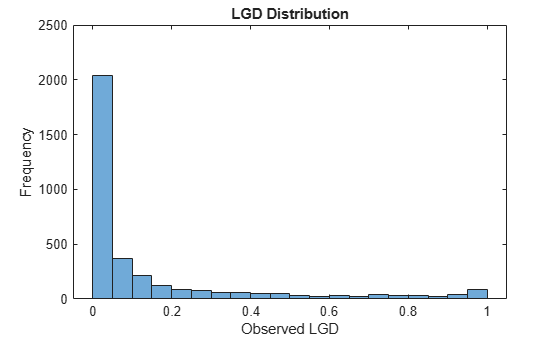Explore the relationships between the predictors and the response. The Spearman correlation between the selected predictor and the LGD is displayed first. The Spearman correlation is one of the rank order statistics commonly used for LGD modeling .

```SelectedPredictor =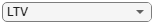'LTV'; fprintf('Spearman correlation between %s and LGD: %g',SelectedPredictor,corr(double(data.(SelectedPredictor)),data.LGD,'Type','Spearman'))```
```Spearman correlation between LTV and LGD: 0.271204 ```
```if isnumeric(data.(SelectedPredictor)) scatter(data.(SelectedPredictor),data.LGD) X = [ones(height(data),1) data.(SelectedPredictor)]; b = X\data.LGD; y = X*b; hold on plot(data.(SelectedPredictor),y) ylim([0 1]) hold off xlabel(SelectedPredictor) ylabel('LGD') end```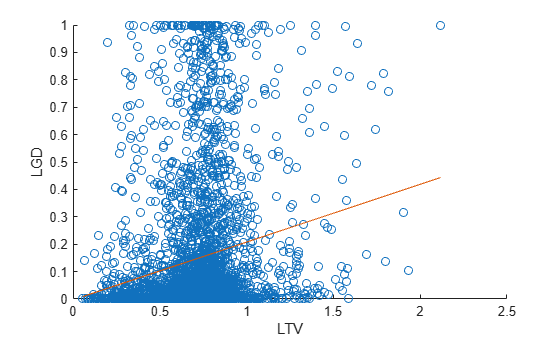For numeric predictors, there is a scatter plot of the LGD against the selected predictor values, with a superimposed linear fit. There is a significant amount of noise in the data, with points scattered all over the plot. This is a common situation for LGD data modeling. The density of the dots is sometimes different in different areas of the plot, suggesting relationships. The slope of the linear fit and the Spearman correlation give more information about the relationship between the selected predictor and the response.

Visually assessing the density of the points in a scatter plot might not be a reliable approach to understand the distribution of the data. To better understand the distribution of LGD values for different levels of a selected predictor, create a box plot.

```% Choose the number of discretization levels for numeric predictors NumLevels =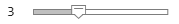3; if isnumeric(data.(SelectedPredictor)) PredictorEdges = linspace(min(data.(SelectedPredictor)),max(data.(SelectedPredictor)),NumLevels+1); PredictorDiscretized = discretize(data.(SelectedPredictor),PredictorEdges,'Categorical',string(PredictorEdges(2:end))); boxplot(data.LGD,PredictorDiscretized) xlabel([SelectedPredictor ' Discretized']) ylabel('LGD') else boxplot(data.LGD,data.(SelectedPredictor)) xlabel(SelectedPredictor) ylabel('LGD') end```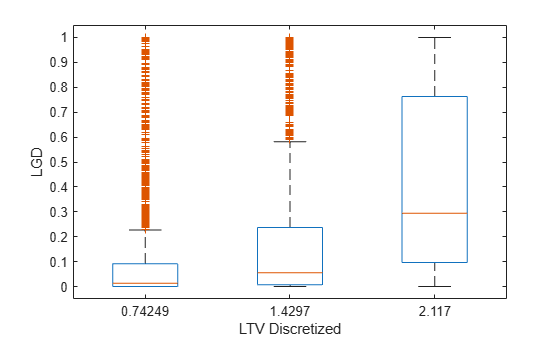For categorical data, the box plot is straightforward since a small number of levels are already given. For numeric data, you can discretize the data first and then generate the box plot. Different box sizes and heights show that the distribution of LGD values changes for different predictor levels. A monotonic trend in the median (red horizontal line in the center of the boxes) shows a potential linear relationship between the predictor and the LGD (though possibly a mild relationship, due to the wide distributions).

### Mean LGD Over Different Groups

The basic approach to predict LGD is to simply use the mean of the LGD data. Although this is a straightforward approach, easy to understand and use, the downside is that the mean is a constant value and this approach sheds no light on the sensitivity of LGD to other risk factors. In particular, the predictors in the data set are ignored.

To introduce sensitivity to predictors, the mean LGD values can be estimated over different groups or segments of the data, where the groups are defined using ranges of the predictor values. This approach is still a relatively straightforward approach, yet it can noticeably reduce the prediction error compared to a single mean LGD value for all observations.

To start, separate the data set into training and testing data. The same training and testing data sets are used for all approaches in this example.

```NumObs = height(data); % Reset the random stream state, for reproducibility % Comment this line out to generate different data partitions each time the example is run rng('default'); c = cvpartition(NumObs,'HoldOut',0.4); TrainingInd = training(c); TestInd = test(c);```

In this example, the groups are defined using the three predictors. `LTV` is discretized into low and high levels. `Age` is discretized into young and old loans. `Type` already has two levels, namely, residential and investment. The groups are all the combinations of these values (for example, low LTV, young loan, residential, and so on).

The number of levels and the specific cut off points are for illustration purposes only, you can base other discretizations on different criteria. Also, using all predictors for the discretization may not be ideal when the data set contains many predictors. In some cases, using a single predictor, or a couple of predictors, may be enough to find useful groups with distinct mean LGD values. When the data includes macro information, the grouping may include a macro variable; for example, the mean LGD value should be different over recessions vs. economic expansions.

Compute the mean LGD over the eight data groups using the training data.

```% Discretize LTV LTVEdges = [0 0.5 max(data.LTV)]; data.LTVDiscretized = discretize(data.LTV,LTVEdges,'Categorical',{'low','high'}); % Discretize Age AgeEdges = [0 2 max(data.Age)]; data.AgeDiscretized = discretize(data.Age,AgeEdges,'Categorical',{'young','old'}); % Find group means on training data gs = groupsummary(data(TrainingInd,:),{'LTVDiscretized','AgeDiscretized','Type'},'mean','LGD'); disp(gs)```
``` LTVDiscretized AgeDiscretized Type GroupCount mean_LGD ______________ ______________ ___________ __________ ________ low young residential 163 0.12166 low young investment 26 0.087331 low old residential 175 0.021776 low old investment 23 0.16379 high young residential 1134 0.16489 high young investment 257 0.25977 high old residential 265 0.066068 high old investment 50 0.11779 ```

For prediction, the test data is mapped into the eight groups, and then the corresponding group mean is set as the predicted LGD value.

```LGDGroupTest = findgroups(data(TestInd,{'LTVDiscretized' 'AgeDiscretized' 'Type'})); LGDPredictedByGroupMeans = gs.mean_LGD(LGDGroupTest);```

Store the observed LGD and the predicted LGD in a new table `dataLGDPredicted`. This table stores predicted LGD values for all other approaches in the example.

```dataLGDPredicted = table; dataLGDPredicted.Observed = data.LGD(TestInd); dataLGDPredicted.GroupMeans = LGDPredictedByGroupMeans; disp(head(dataLGDPredicted))```
``` Observed GroupMeans _________ __________ 0.0064766 0.066068 0.007947 0.12166 0.063182 0.25977 0 0.066068 0.10904 0.16489 0 0.16489 0.89463 0.16489 0 0.021776 ```

The Model Comparison section has a more detailed comparison of all models that includes visualizations and prediction error metrics.

### Simple Regression Model

A natural approach is to use a regression model to explicitly model a relationship between the LGD and some predictors. LGD data, however, is bounded in the unit interval, whereas the response variable for linear regression models is, in theory, unbounded.

To apply simple linear regression approaches, the LGD data can be transformed. A common transformation is the logit function, which leads to the following regression model:

`$\mathrm{log}\left(\frac{\mathrm{LGD}}{1-\mathrm{LGD}}\right)=\mathit{X}\beta +ϵ,\mathrm{with}\text{\hspace{0.17em}}ϵ~\mathit{N}\left(0,{\sigma }^{2}\right)$`

LGD values of `0` or `1` cause the logit function to take infinite values, so the LGD data is typically truncated before applying the transformation.

```data.LGDTruncated = data.LGD; data.LGDTruncated(data.LGD==0) = 0.00001; data.LGDTruncated(data.LGD==1) = 0.99999; data.LGDLogit = log(data.LGDTruncated./(1-data.LGDTruncated));```

Below is the histogram of the transformed LGD data that uses the logit function. The range of values spans positive and negative values, which is consistent with the linear regression requirements. The distribution still shows significant mass probability points at the ends of the distribution.

```histogram(data.LGDLogit) title('Logit Transformation of Truncated LGD Data')```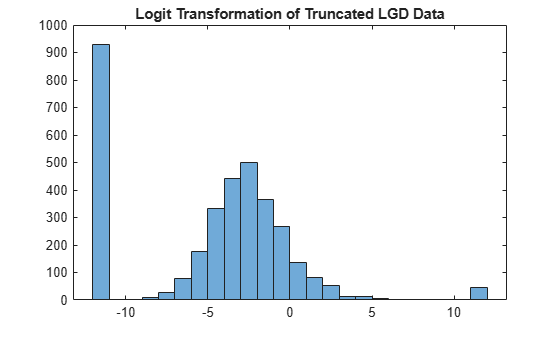Other transformations are suggested in the literature . For example, instead of the logit function, the truncated LGD values can be mapped with the inverse standard normal distribution (similar to a probit model).

Fit a regression model using the `fitLGDModel` function from Risk Management Toolbox™ using the training data. By default, a logit transformation is applied to the LGD response data with a boundary tolerance of `1e-5`. For more information on the supported transformations and optional arguments, see `Regression`.

```mdlRegression = fitLGDModel(data(TrainingInd,:),'regression','PredictorVars',{'LTV' 'Age' 'Type'},'ResponseVar','LGD'); disp(mdlRegression)```
``` Regression with properties: ResponseTransform: "logit" BoundaryTolerance: 1.0000e-05 ModelID: "Regression" Description: "" UnderlyingModel: [1x1 classreg.regr.CompactLinearModel] PredictorVars: ["LTV" "Age" "Type"] ResponseVar: "LGD" ```
`disp(mdlRegression.UnderlyingModel)`
```Compact linear regression model: LGD_logit ~ 1 + LTV + Age + Type Estimated Coefficients: Estimate SE tStat pValue ________ ________ _______ __________ (Intercept) -4.7549 0.36041 -13.193 3.0997e-38 LTV 2.8565 0.41777 6.8377 1.0531e-11 Age -1.5397 0.085716 -17.963 3.3172e-67 Type_investment 1.4358 0.2475 5.8012 7.587e-09 Number of observations: 2093, Error degrees of freedom: 2089 Root Mean Squared Error: 4.24 R-squared: 0.206, Adjusted R-Squared: 0.205 F-statistic vs. constant model: 181, p-value = 2.42e-104 ```

The model coefficients match the findings in the exploratory data analysis, with a positive coefficient for `LTV`, a negative coefficient for `Age`, and a positive coefficient for investment properties in the `Type` variable.

The `Regression` LGD models support prediction and apply the inverse transformation so the predicted LGD values are in the LGD scale. For example, for the model fitted above that uses the logit transformation, the inverse logit transformation (also known as the logistic, or sigmoid function) is applied by the predict function to return an LGD predicted value.

```dataLGDPredicted.Regression = predict(mdlRegression,data(TestInd,:)); disp(head(dataLGDPredicted))```
``` Observed GroupMeans Regression _________ __________ __________ 0.0064766 0.066068 0.00091169 0.007947 0.12166 0.0036758 0.063182 0.25977 0.18774 0 0.066068 0.0010877 0.10904 0.16489 0.011213 0 0.16489 0.041992 0.89463 0.16489 0.052947 0 0.021776 3.7188e-06 ```

The Model Comparison section at the end of this example has a more detailed comparison of all models that includes visualizations and prediction error metrics. In particular, the histogram of the predicted LGD values shows that the regression model predicts many LGD values near zero, even though the high probability near zero was not explicitly modeled.

### Tobit Regression Model

Tobit or censored regression is designed for models where the response is bounded. The idea is that there is an underlying (latent) linear model but that the observed response values, in this case the LGD values, are truncated. For this example, use a model censored on both sides with a left limit at `0` and a right limit at `1`, corresponding to the following model formula

`${\mathrm{LGD}}_{\mathit{i}}=\mathrm{min}\left(\mathrm{max}\left(0,{{\mathit{Y}}_{\mathit{i}}}^{*}\right),1\right)$`

with:

`$\begin{array}{l}{{\mathit{Y}}_{\mathit{i}}}^{*}={\mathit{X}}_{\mathit{i}}\beta +{ϵ}_{\mathit{i}}\\ ={\beta }_{0\text{\hspace{0.17em}}}+{\beta }_{1}{{\mathit{X}}^{1}}_{\mathit{i}}+\cdots +{\beta }_{\mathit{k}}{{\mathit{X}}^{\mathit{k}}}_{\mathit{i}}+{ϵ}_{\mathit{i}}\text{\hspace{0.17em}},\\ \mathrm{with}\text{\hspace{0.17em}}{ϵ}_{\mathit{i}}~\mathit{N}\left(0,{\sigma }^{2}\right)\end{array}$`

The model parameters are all the $\beta$s and the standard deviation of the error $\sigma$.

Fit the Tobit regression model with `fitLGDModel` using the training data. By default, a model censored on both sides is fitted with limits at `0` and `1`. For more information on Tobit models, see `Tobit`.

```mdlTobit = fitLGDModel(data(TrainingInd,:),'tobit','CensoringSide','both','PredictorVars',{'LTV' 'Age' 'Type'},'ResponseVar','LGD'); disp(mdlTobit)```
``` Tobit with properties: CensoringSide: "both" LeftLimit: 0 RightLimit: 1 ModelID: "Tobit" Description: "" UnderlyingModel: [1x1 risk.internal.credit.TobitModel] PredictorVars: ["LTV" "Age" "Type"] ResponseVar: "LGD" ```
`disp(mdlTobit.UnderlyingModel)`
```Tobit regression model: LGD = max(0,min(Y*,1)) Y* ~ 1 + LTV + Age + Type Estimated coefficients: Estimate SE tStat pValue _________ _________ _______ __________ (Intercept) 0.058257 0.027279 2.1356 0.032828 LTV 0.20126 0.031383 6.4129 1.7592e-10 Age -0.095407 0.0072435 -13.171 0 Type_investment 0.10208 0.018054 5.6544 1.7785e-08 (Sigma) 0.29288 0.0057071 51.318 0 Number of observations: 2093 Number of left-censored observations: 547 Number of uncensored observations: 1521 Number of right-censored observations: 25 Log-likelihood: -698.383 ```

Tobit models predict using the unconditional expected value of the response, given the predictor values. For more information, see Loss Given Default Tobit Models.

```dataLGDPredicted.Tobit = predict(mdlTobit,data(TestInd,:)); disp(head(dataLGDPredicted))```
``` Observed GroupMeans Regression Tobit _________ __________ __________ ________ 0.0064766 0.066068 0.00091169 0.087889 0.007947 0.12166 0.0036758 0.12432 0.063182 0.25977 0.18774 0.32043 0 0.066068 0.0010877 0.093354 0.10904 0.16489 0.011213 0.16718 0 0.16489 0.041992 0.22382 0.89463 0.16489 0.052947 0.23695 0 0.021776 3.7188e-06 0.010234 ```

The Model Comparison section at the end of this example has a more detailed comparison of all models that includes visualizations and prediction error with different metrics. The histogram of the predicted LGD values for the Tobit model does not have a U-shaped distribution, but it ranks well compared to other models.

### Beta Regression Model

In a Beta regression model for LGD, the model does not directly predict a single LGD value, it predicts an entire distribution of LGDs (given the predictor values). From that distribution, a value must be determined to predict a single LGD value for a loan, typically the mean of that distribution.

Technically, given the predictor values ${\mathit{X}}_{1},{\mathit{X}}_{2,...}$ and model coefficients $\mathit{b}$ and $\mathit{c}$, you can:

• Compute values for the parameters $\mu \text{\hspace{0.17em}}$(mean) and $\varphi \text{\hspace{0.17em}}$(precision, sometimes called the "sample size" of the beta distribution with the following formulas:

`$\begin{array}{l}\mu =\frac{1}{1+\mathrm{exp}\left({-\mathit{b}}_{0}-{\mathit{b}}_{1}{\mathit{X}}_{1}-\cdots \right)}\\ \varphi =\mathrm{exp}\left({\mathit{c}}_{0}+{\mathit{c}}_{1}{\mathit{X}}_{1}+\cdots \text{\hspace{0.17em}}\right)\end{array}$`

• Compute values for $\alpha \text{\hspace{0.17em}}$and $\beta$, the typical parameters of the beta distribution, with these formulas:

`$\alpha =\mu \text{\hspace{0.17em}}\varphi \text{\hspace{0.17em}}$`

`$\beta =\left(1-\mu \text{\hspace{0.17em}}\right)\text{\hspace{0.17em}}\varphi \text{\hspace{0.17em}}$`

• Evaluate the density function of the corresponding beta distribution for a given level of $\mathrm{LGD}$, where $\Gamma$ is the gamma function; see  for details:

`${\mathit{f}}_{\mathrm{beta}}\left(\mathrm{LGD}|\alpha \text{\hspace{0.17em}},\beta \text{\hspace{0.17em}}\right)=\frac{\Gamma \text{\hspace{0.17em}}\left(\alpha +\beta \text{\hspace{0.17em}}\right)}{\Gamma \text{\hspace{0.17em}}\left(\alpha \text{\hspace{0.17em}}\right)\Gamma \text{\hspace{0.17em}}\left(\beta \text{\hspace{0.17em}}\right)}{\mathrm{LGD}}^{\alpha -1}{\left(1-\mathrm{LGD}\right)}^{\beta -1}$`

For fitting the model, once the density function is evaluated, you can update the likelihood function and find the optimal coefficients with a maximum likelihood approach.

For prediction, once the model coefficients are fit, a prediction can be made, typically using the mean of the distribution, that is, the $\mu$ parameter, as the predicted LGD value.

Fit a Beta regression model using the `fitLGDModel` function from Risk Management Toolbox™ using the training data. By default, a boundary tolerance of `1e-5 `is applied for the LGD response data. For more information on Beta models, see `Beta`.

```mdlBeta = fitLGDModel(data(TrainingInd,:),'beta','PredictorVars',{'LTV' 'Age' 'Type'},'ResponseVar','LGD'); disp(mdlBeta)```
``` Beta with properties: BoundaryTolerance: 1.0000e-05 ModelID: "Beta" Description: "" UnderlyingModel: [1x1 risk.internal.credit.BetaModel] PredictorVars: ["LTV" "Age" "Type"] ResponseVar: "LGD" ```
`disp(mdlBeta.UnderlyingModel)`
```Beta regression model: logit(LGD) ~ 1_mu + LTV_mu + Age_mu + Type_mu log(LGD) ~ 1_phi + LTV_phi + Age_phi + Type_phi Estimated coefficients: Estimate SE tStat pValue ________ ________ _______ __________ (Intercept)_mu -1.3772 0.13201 -10.433 0 LTV_mu 0.60269 0.15087 3.9947 6.7023e-05 Age_mu -0.47464 0.040264 -11.788 0 Type_investment_mu 0.45372 0.085143 5.3289 1.094e-07 (Intercept)_phi -0.16337 0.12591 -1.2975 0.19462 LTV_phi 0.055892 0.14719 0.37973 0.70419 Age_phi 0.22887 0.040335 5.6743 1.5863e-08 Type_investment_phi -0.14102 0.078155 -1.8044 0.071311 Number of observations: 2093 Log-likelihood: -5291.04 ```

For prediction, recall that the beta regression links the predictors to an entire beta distribution. For example, suppose that a loan has an `LTV` of `0.7` and an Age of `1.1` years, and it is an investment property. The beta regression model gives us a prediction for the $\alpha \text{\hspace{0.17em}}$and $\beta \text{\hspace{0.17em}}$parameters for this loan, and the model predicts that for this loan the range of possible LGD values follows the corresponding beta distribution.

```Estimate = mdlBeta.UnderlyingModel.Coefficients.Estimate; NumCols = mdlBeta.UnderlyingModel.NumCoefficients/2; XExample = [1 0.7 1.1 1]; MuExample = 1/(1+exp(-XExample*Estimate(1:NumCols))); NuExample = exp(XExample*Estimate(NumCols+1:end)); AlphaExample = MuExample*NuExample; BetaExample = (1-MuExample)*NuExample; xDomain = 0.01:0.01:0.99; pBeta = betapdf(xDomain,AlphaExample,BetaExample); plot(xDomain,pBeta) title('Predicted Distribution, Single Loan') xlabel('Possible LGD') ylabel('Predicted Density')```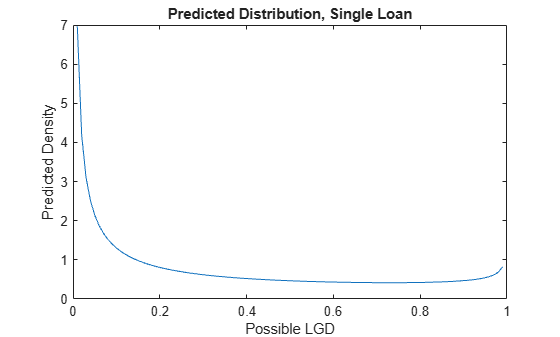The shape of the distribution has the U-shaped pattern of the data. However, this is a predicted distribution of LGD values for a single loan. This distribution can be very useful for simulation purposes. However, to predict an LGD value for this loan, a method is required that goes from an entire distribution to a single value.

One way to predict would be to randomly draw a value from the previous distribution. This would tend to give predicted values towards the ends of the unit interval, and the overall shape of the distribution for a data set would match the U-shaped patter of observed LGD values. However, even if the shape of the distribution looked correct, a random draw from the distribution does not work well for prediction purposes. Two points with the same predictor values would have two different predicted LGDs, which is counterintuitive. Moreover, the prediction error at the observation level could be large, since many loans with small observed LGDs could get random predictions of large LGDs, and vice versa.

To reduce the prediction error at the individual level, the expected value of the beta distribution is typically used to predict. The distribution of predicted values with this approach does not have the expected U-shaped pattern because the mean value tends to be away from the boundaries of the unit interval. However, by using the mean of the beta distribution, all observations with the same predictor values get the same predicted LGDs. Moreover, the mean may not be close to values that are on the ends of the distribution, but the average error might be smaller compared to the random draws from the previous approach.

Use `predict` with Beta models to predict using the mean of the beta distribution. Remember that the expected value of the distribution is the $\mu \text{\hspace{0.17em}}$parameter, so the mean value prediction is straightforward.

```dataLGDPredicted.Beta = predict(mdlBeta,data(TestInd,:)); disp(head(dataLGDPredicted))```
``` Observed GroupMeans Regression Tobit Beta _________ __________ __________ ________ ________ 0.0064766 0.066068 0.00091169 0.087889 0.093695 0.007947 0.12166 0.0036758 0.12432 0.14915 0.063182 0.25977 0.18774 0.32043 0.35263 0 0.066068 0.0010877 0.093354 0.096434 0.10904 0.16489 0.011213 0.16718 0.18858 0 0.16489 0.041992 0.22382 0.2595 0.89463 0.16489 0.052947 0.23695 0.26767 0 0.021776 3.7188e-06 0.010234 0.021315 ```

The Model Comparison section at the end of this example has a more detailed comparison of all models that includes visualizations and prediction error metrics. In particular, the histogram of the predicted LGD values shows that the beta regression approach does not produce a U-shaped distribution. However, this approach does have good performance under the other metrics reported.

### Two-Stage Model

Two-stage LGD models separate the case with no losses (LGD equal to `0`) from the cases with actual losses (LGD greater than `0`) and build two models. The stage 1 model is a classification model to predict whether the loan will have positive LGD. The stage 2 model a regression-type model to predict the actual LGD when the LGD is expected to be positive. The prediction is the expected value of the two combined models, which is the product of the probability of having a loss (stage 1 prediction) times the expected LGD value (stage 2 prediction).

In this example, a logistic regression model is used for the stage 1. Stage two is a regression on a logit transformation of the positive LGD data, fitted using `fitLGDModel`. More sophisticated models can be used for stage 1 and stage 2 models, see for example  or . Also, another extension is to explicitly handle the LGD = `1` boundary. The stage 1 model would produce probabilities of observing an LGD of `0`, an LGD of `1`, and an LGD value strictly between `0` and `1`. The stage 2 model would predict LGD values when the LGD is expected to be strictly between `0` and `1`.

Use `fitglm` to fit the stage 1 model using the training data. The response variable is an indicator with a value of `1` if the observed LGD is positive, and `0` if the observed LGD is zero.

```IndLGDPositive = data.LGD>0; data.LGDPositive = IndLGDPositive; disp(head(data))```
``` LTV Age Type LGD LTVDiscretized AgeDiscretized LGDTruncated LGDLogit LGDPositive _______ _______ ___________ _________ ______________ ______________ ____________ ________ ___________ 0.89101 0.39716 residential 0.032659 high young 0.032659 -3.3884 true 0.70176 2.0939 residential 0.43564 high old 0.43564 -0.25887 true 0.72078 2.7948 residential 0.0064766 high old 0.0064766 -5.0331 true 0.37013 1.237 residential 0.007947 low young 0.007947 -4.827 true 0.36492 2.5818 residential 0 low old 1e-05 -11.513 false 0.796 1.5957 residential 0.14572 high young 0.14572 -1.7686 true 0.60203 1.1599 residential 0.025688 high young 0.025688 -3.6357 true 0.92005 0.50253 investment 0.063182 high young 0.063182 -2.6965 true ```
```mdl1 = fitglm(data(TrainingInd,:),"LGDPositive ~ 1 + LTV + Age + Type",'Distribution',"binomial"); disp(mdl1)```
```Generalized linear regression model: logit(LGDPositive) ~ 1 + LTV + Age + Type Distribution = Binomial Estimated Coefficients: Estimate SE tStat pValue ________ ________ _______ __________ (Intercept) 1.3157 0.21193 6.2083 5.3551e-10 LTV 1.3159 0.25328 5.1954 2.0433e-07 Age -0.79597 0.053607 -14.848 7.1323e-50 Type_investment 0.66784 0.17019 3.9241 8.7051e-05 2093 observations, 2089 error degrees of freedom Dispersion: 1 Chi^2-statistic vs. constant model: 404, p-value = 2.68e-87 ```

A receiver operating characteristic (ROC) curve plot for the stage 1 model is commonly reported using test data, as well as the area under the ROC curve (AUROC or simply AUC).

```PredictedProbLGDPositive = predict(mdl1,data(TestInd,:)); [x,y,~,AUC] = perfcurve(data.LGDPositive(TestInd),PredictedProbLGDPositive,1); plot(x,y) title(sprintf('ROC Stage 1 Model (AUROC: %g)',AUC))```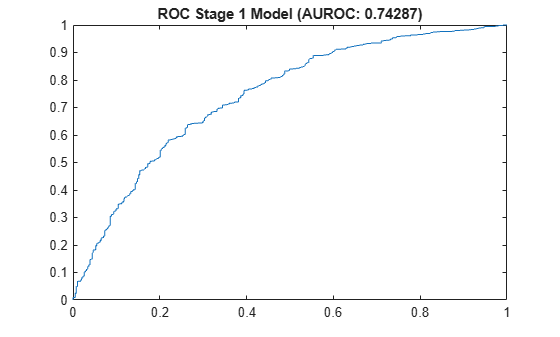Fit the stage 2 model with `fitLGDModel` using only the training data with a positive LGD. This is the same type of model used earlier in the Regression section, however, this time it is fitted using only observations from the training data with positive LGDs.

```dataLGDPositive = data(TrainingInd&IndLGDPositive,{'LTV','Age','Type','LGD'}); mdl2 = fitLGDModel(dataLGDPositive,'regression'); disp(mdl2.UnderlyingModel)```
```Compact linear regression model: LGD_logit ~ 1 + LTV + Age + Type Estimated Coefficients: Estimate SE tStat pValue ________ ________ _______ __________ (Intercept) -2.9083 0.27538 -10.561 3.2039e-25 LTV 1.3883 0.31838 4.3604 1.384e-05 Age -0.46116 0.081939 -5.6281 2.1608e-08 Type_investment 0.78223 0.18096 4.3226 1.6407e-05 Number of observations: 1546, Error degrees of freedom: 1542 Root Mean Squared Error: 2.8 R-squared: 0.0521, Adjusted R-Squared: 0.0503 F-statistic vs. constant model: 28.3, p-value = 8.73e-18 ```

Perform prediction on the test data using the combined models for stage 1 and stage 2. The predicted LGD is the product of the probability of observing a positive LGD from the stage 1 model times the expected LGD value predicted by the stage 2 model. Recall that regression models fitted using `fitLGDModel` apply the inverse transformation during prediction, so the predicted value is a valid LGD value.

```PredictedLGDPositive = predict(mdl2,data(TestInd,:)); % PredictedProbLGDPositive is computed before the ROC curve above % Final LGD prediction is the product of stage 1 and stage 2 predictions dataLGDPredicted.TwoStage = PredictedProbLGDPositive.*PredictedLGDPositive; disp(head(dataLGDPredicted))```
``` Observed GroupMeans Regression Tobit Beta TwoStage _________ __________ __________ ________ ________ _________ 0.0064766 0.066068 0.00091169 0.087889 0.093695 0.020038 0.007947 0.12166 0.0036758 0.12432 0.14915 0.034025 0.063182 0.25977 0.18774 0.32043 0.35263 0.2388 0 0.066068 0.0010877 0.093354 0.096434 0.022818 0.10904 0.16489 0.011213 0.16718 0.18858 0.060072 0 0.16489 0.041992 0.22382 0.2595 0.097685 0.89463 0.16489 0.052947 0.23695 0.26767 0.11142 0 0.021776 3.7188e-06 0.010234 0.021315 0.0003689 ```

The Model Comparison section at the end of this example has a more detailed comparison of all models that includes visualizations and prediction error metrics. This approach also ranks well compared to other models and the histogram of the predicted LGD values shows high frequencies near `0`.

### Model Comparison

To evaluate the performance of LGD models, different metrics are commonly used. One metric is the R-squared of the linear fit regressing the observed LGD values on the predicted values. A second metric is some correlation or rank order statistic; this example uses the Spearman correlation. For prediction error, root mean squared error (RMSE) is a common metric. Also, a simple metric sometimes reported is the difference between the mean LGD value in the data and the mean LGD value of the predictions.

The Regression, Tobit, and Beta models directly support these metrics with the `modelCalibration` function, including comparing against a reference model. For example, here is a report of these metrics for the regression model, passing the predictions of the simple nonparametric model as reference.

```CalMeasure = modelCalibration(mdlRegression,data(TestInd,:),'DataID','Test','ReferenceLGD',dataLGDPredicted.GroupMeans,'ReferenceID','Group Means','CorrelationType','spearman'); disp(CalMeasure)```
``` RSquared RMSE Correlation SampleMeanError ________ _______ ___________ _______________ Regression, Test 0.070867 0.25988 0.42152 0.10759 Group Means, Test 0.041622 0.2406 0.33807 -0.0078124 ```

A visualization is also directly supported with `modelCalibrationPlot`.

`modelCalibrationPlot(mdlRegression,data(TestInd,:),'DataID','Test','ReferenceLGD',dataLGDPredicted.GroupMeans,'ReferenceID','Group Means')`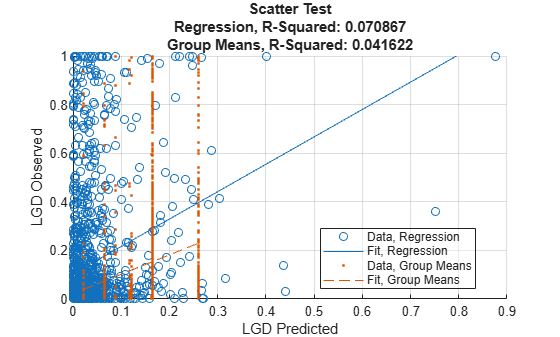In addition, `Regression`, `Tobit` and `Beta` models support model discrimination tools, with the `modelDiscrimination` and `modelDiscriminationPlot` functions. For model discrimination, the LGD data is discretized (high LGD vs. low LGD) and the ROC curve and the corresponding AUROC are computed in a standard way. For more information, see `modelDiscrimination` and `modelDiscriminationPlot`. For example, here is the ROC curve for the regression model, with the ROC curve of the nonparametric model as reference.

`modelDiscriminationPlot(mdlRegression,data(TestInd,:),'DataID','Test','ReferenceLGD',dataLGDPredicted.GroupMeans,'ReferenceID','Group Means')`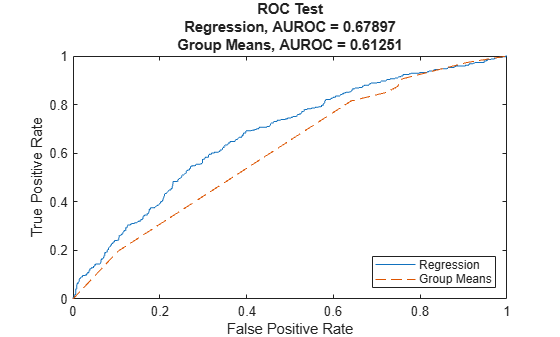The rest of this model validation section works with the predicted LGD values from all the models to compute the metrics mentioned above (R-squared, Spearman correlation, RMSE and sample mean error). It also shows a scatter plot, a histogram, and a box plot to further analyze the performance of the models.

The four metrics are reported below, sorted by decreasing R-squared values.

```ModelNames = dataLGDPredicted.Properties.VariableNames(2:end); % Remove 'Observed' NumModels = length(ModelNames); SampleMeanError = zeros(NumModels,1); RSquared = zeros(NumModels,1); Spearman = zeros(NumModels,1); RMSE = zeros(NumModels,1); lmAll = struct; meanLGDTest = mean(dataLGDPredicted.Observed); for ii=1:NumModels % R-squared, and store linear model fit for visualization section Formula = ['Observed ~ 1 + ' ModelNames{ii}]; lmAll.(ModelNames{ii}) = fitlm(dataLGDPredicted,Formula); RSquared(ii) = lmAll.(ModelNames{ii}).Rsquared.Ordinary; % Spearman correlation Spearman(ii) = corr(dataLGDPredicted.Observed,dataLGDPredicted.(ModelNames{ii}),'type','Spearman'); % Root mean square error RMSE(ii) = sqrt(mean((dataLGDPredicted.Observed-dataLGDPredicted.(ModelNames{ii})).^2)); % Sample mean error SampleMeanError(ii) = meanLGDTest-mean(dataLGDPredicted.(ModelNames{ii})); end PerformanceMetrics = table(RSquared,Spearman,RMSE,SampleMeanError,'RowNames',ModelNames); PerformanceMetrics = sortrows(PerformanceMetrics,'RSquared','descend'); disp(PerformanceMetrics)```
``` RSquared Spearman RMSE SampleMeanError ________ ________ _______ _______________ TwoStage 0.090814 0.41987 0.24197 0.060619 Tobit 0.08527 0.42217 0.23712 -0.034412 Beta 0.080804 0.41557 0.24112 -0.052396 Regression 0.070867 0.42152 0.25988 0.10759 GroupMeans 0.041622 0.33807 0.2406 -0.0078124 ```

For the particular training vs. test partition used in this example, the two-stage model has the highest R-squared, although for other partitions, Tobit has the highest R-squared value. Even though the group means approach does not have a high R-squared value, it typically has the smallest sample mean error (mean of predicted LGD values minus mean LGD in the test data). The group means are also competitive for the RMSE metric.

Report the model performance one approach at a time, including visualizations. Display the metrics for the selected model.

```ModelSelected =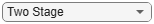"TwoStage"; disp(PerformanceMetrics(ModelSelected,:))```
``` RSquared Spearman RMSE SampleMeanError ________ ________ _______ _______________ TwoStage 0.090814 0.41987 0.24197 0.060619 ```

Plot the regression fit (observed LGD vs. predicted LGD), which is a common visual tool to assess the model performance. This is essentially the same visualization as the `modelCalibrationPlot` function shown above, but using the `plot` function of the fitted linear models. The R-squared reported above is the R-squared of this regression. The plot shows a significant amount of error for all models. A good predictive model would have the points located mostly along the diagonal, and not be scattered all over the unit square. However, the metrics above do show some differences in predictive performance for different models that can be important in practice.

```plot(lmAll.(ModelSelected)) xlim([0 1]) ylim([0 1])```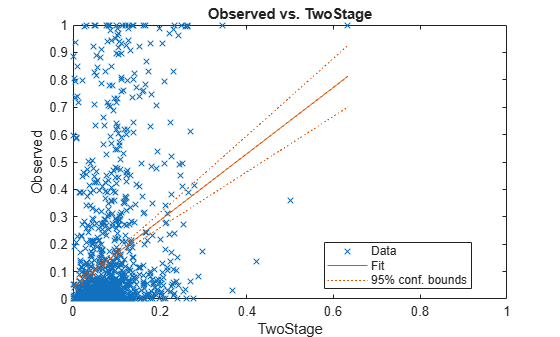Compare the histograms of the predicted and observed LGD values. For some models, the distribution of predicted values shows high frequencies near zero, similar to the U shape of the observed LGD distribution. However, matching the shape of the distribution does not mean high accuracy at the level of individual predictions; some models show better prediction error even though their histogram does not have a U shape.

```LGDEdges = 0:0.1:1; % Ten bins to better show the distribution shape y1 = histcounts(dataLGDPredicted.(ModelSelected),LGDEdges); y2 = histcounts(dataLGDPredicted.Observed,LGDEdges); bar((LGDEdges(1:end-1)+LGDEdges(2:end))/2,[y1; y2]) title(strcat(ModelSelected,' Model')) ylabel('Frequency') xlabel('LGD') legend('Predicted','Observed') grid on```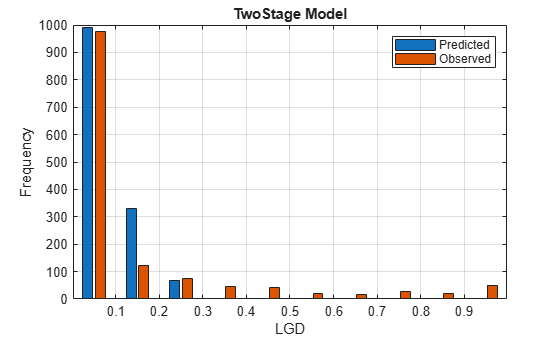Show the box plot of the observed LGD values for different ranges of predicted LGD values. A coarser discretization (five bins only) smooths some noise out and better summarizes the underlying relationship. Ideally, the median (red horizontal line in the middle) should have a monotonic trend and be clearly different from one level to the next. Tall boxes also mean that there is a significant amount of error around the predicted values, which in some cases may be due to very few observations in that level. For a good predictive model, the boxes should be short and be located near the diagonal as you move from one level to the next.

```LGDEdges = linspace(min(dataLGDPredicted.(ModelSelected)),max(dataLGDPredicted.(ModelSelected)),6); % Five bins LGDDiscretized = discretize(dataLGDPredicted.(ModelSelected),LGDEdges,'Categorical',string(LGDEdges(2:end))); boxplot(dataLGDPredicted.Observed,LGDDiscretized) ylim([0 1]) title(strcat(ModelSelected,' Model')) xlabel('Predicted LGD, Discretized') ylabel('Observed LGD')```### Summary

This example shows multiple approaches for LGD modeling and prediction. The Regression, Tobit, and Beta models (including the regression model of the second stage in the two-stage model) are fitted using the `fitLGDModel` function from Risk Management Toolbox.

The workflow in this example can be adapted to further analyze the models discussed here or to implement and validate other modeling approaches. This example can be extended to perform a more thorough comparison of LGD models (see for example  and ).

The example can also be extended to perform a cross-validation analysis to either benchmark alternative models or to fine-tune hyperparameters. For example, better cut off points for the group means could be selected using cross-validation, or alternative transformations of the LGD response values (logit, probit) could be benchmarked to select the one with the best performance. This example can also be a starting point to perform a backtesting analysis using out-of-time data; see for example .

### References

 Baesens, B., D. Rosch, and H. Scheule. Credit Risk Analytics. Wiley, 2016.

 Johnston Ross, E., and L. Shibut. "What Drives Loss Given Default? Evidence from Commercial Real Estate Loans at Failed Banks." Federal Deposit Insurance Corporation, Center for Financial Research, Working Paper 2015-03, March 2015.

 Li, P., X. Zhang, and X. Zhao. "Modeling Loss Given Default. "Federal Deposit Insurance Corporation, Center for Financial Research, Working Paper 2018-03, July 2018.

 Loterman, G., I. Brown, D. Martens, C. Mues, and B. Baesens. "Benchmarking Regression Algorithms for Loss Given Default Modeling." International Journal of Forecasting. Vol. 28, No.1, pp. 161–170, 2012.

 Loterman, G., M. Debruyne, K. Vanden Branden, T. Van Gestel, and C. Mues. "A Proposed Framework for Backtesting Loss Given Default Models." Journal of Risk Model Validation. Vol. 8, No. 1, pp. 69-90, March 2014.

 Tanoue, Y., and S. Yamashita. "Loss Given Default Estimation: A Two-Stage Model with Classification Tree-Based Boosting and Support Vector Logistic Regression." Journal of Risk. Vol. 21 No. 4, pp. 19-37, 2019.